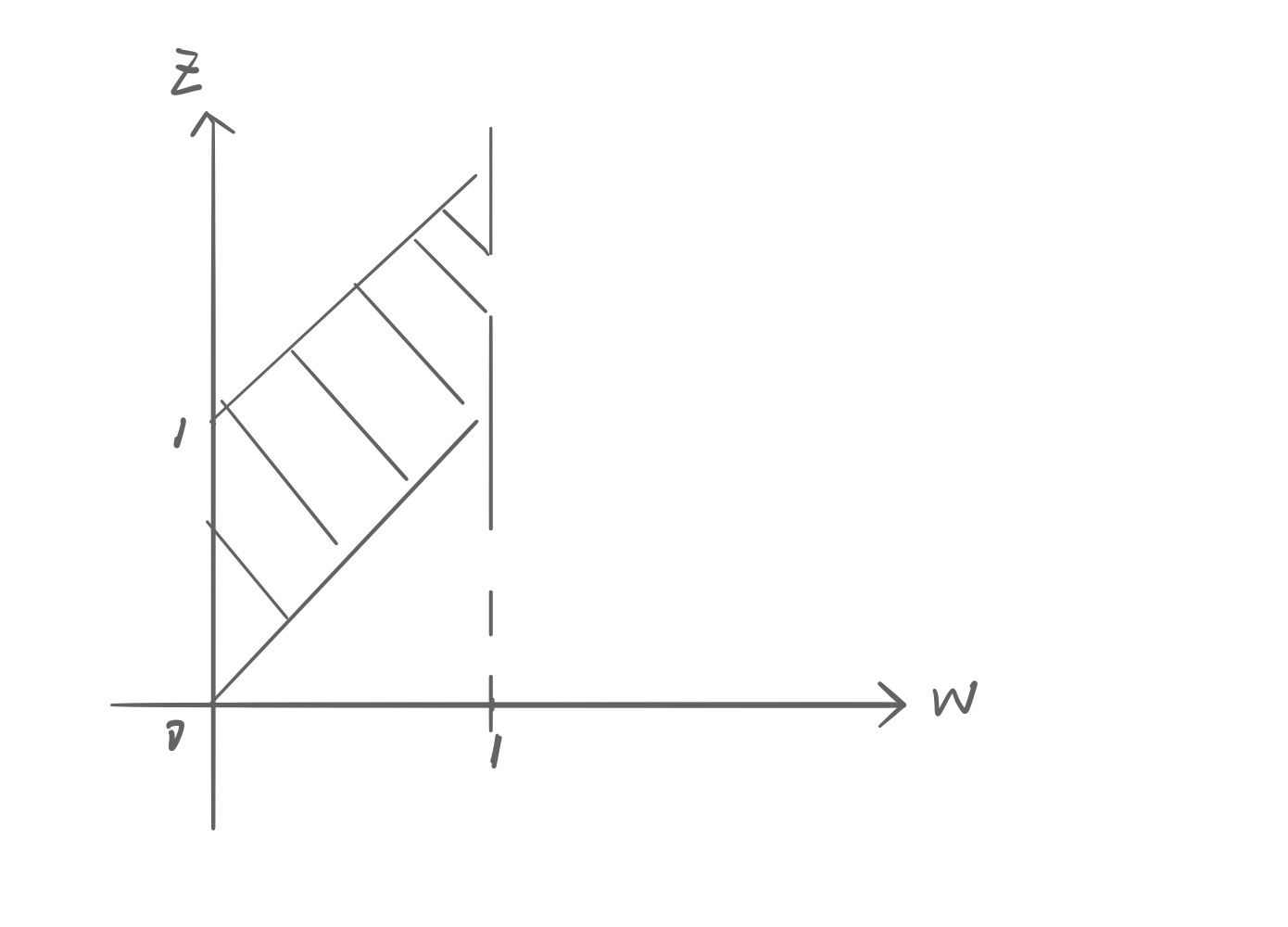• ( 概率论与数理统计教程 第二版 (魏宗舒 著) 高等教育出版社 课后答案.pdf )
• 概率论与数理统计教程的习题答案。《概率论与数理统计教程》主要内容包括事件概率、离散型随机变量、连续型随机变量、大数定律中心极限定理、数理统计的基本概念、点估计、假设检验、方差分析、回归分析、Excel...
• 概率论与数理统计教程课件 高等教育出版社 茆诗松 程依明
• 概率论与数理统计教程（茆诗松·第二版），字数补丁。
• 概率论与数理统计教程+习题解答(茆诗松着) 一共两本书
• 概率论与数理统计教程课后习题答案（茆诗松·第二版）
•sda
• 概率论详细课件/ppt/知识点涵盖详细/《概率论与数理统计教程》第四版/中央财经大学统计数学学院/详细完整/统计、数学专业必备
• 茆诗松《概率论与数理统计教程》（第2版）笔记和课后习题（含考研真题）详解
• 概率论与数理统计教程(第2版) 茆诗松 pdf 习题全解，包含了书中的所有的习题和解答。
• 概率论与数理统计教程_第二版_茆诗松+习题答案+PPT.rar
• 概率论与数理统计教程答案(魏宗舒版)课后习题答案
• 概率论与数理统计教程》习题解答_10830638
• 对应教材 概率论与数理统计教程 对应教材出版社 高等教育出版社 对应教材作者 魏宗舒
• 魏宗舒版的《概率论与数理统计教程》习题答案、课件
• 本文主要讨论《概率论与数理统计教程》中一些比较有趣的知识点。

概率的定义

随机现象的所有可能结果构成样本空间$\Omega = \{ \omega \}$，其中$\omega$称为样本点，认识随机现象需要首先列出样本空间。
设$\mathcal{F}$为$\Omega$的某些子集构成的集合类，且满足：$(1)\Omega \in \mathcal{F}\\ (2)若A\in\mathcal{F}，则\bar{A}\in\mathcal{F}\\ (3)若A_n\in\mathcal{F}，则可列并\bigcup_{n=1}^{\infty}A_n \in \mathcal{F}$则称$\mathcal{F}$为一个事件域，或$\sigma$代数。将$(\Omega, \mathcal{F})$称为可测空间，可测空间中的每个集合都是有概率而言的事件。
设$\Omega$为样本空间，$\mathcal{F}$为事件域，对任意事件$A\in \mathcal{F}$，若定义在$\mathcal{F}$上的实值函数$P(A)$满足：$（1）非负性公理：P(A) > 0\\ （2）正则性公理：P(\Omega) = 1 \\ （3）可列可加性定理：若A_1,A_2,\cdots,A_n互不相容，则P(\bigcup_{i=1}^{\infty}A_i) = \sum_{i=1}^{\infty} P(A_i)$，则称$P(A)$为$A$的概率，将$(\Omega,\mathcal{F},P)$称为概率空间。

由此则得到了概率的公理化定义：概率是建立在样本空间的某些特定子集上的、满足特定条件的实值函数。
2. 确定概率的古典方法

古典方法是建立在经验事实基础上，对被考察事件的可能性进行逻辑分析后得出的该事件的结论。
古典方法定义的概率需要满足：
（1）所涉及的随机现象只有有限种结果。
（2）每个样本点发生的可能性相等。
（3）若事件A中包含k个样本点，则事件A的概率为：$P(A)=\frac{k}{n}$

其中尤其需要注意定义（2），当样本点发生的可能性不等时，是不能直接计算概率的。例如二孩悖论中讨论的：若取基本事件为$\Omega = \{两个男孩，两个女孩，一男一女\}$，则其不符合定义，因为”两个女孩“发生的概率为$1/2$

为什么要研究概率分布

概率分布是全面地、动态地描述随机变量取值的概率规律，从中可以提取有关随机变量的信息，研究更深层次的问题。

随机变量的定义

将定义在样本空间$\Omega$上的实值函数$X=X(\omega)$称为随机变量
用随机变量代表事件：若$B$为实数组成的集合，则$\{X\in B\}$表示随机事件：$\{ \omega:X(\omega) \in B\} \subseteq \Omega$
设$X$为随机变量，$x$为任意实数，称$F(x) = P(X \le x)$为随机变量$X$的分布函数

总结一下，我们有以下映射关系：
$P: \mathcal{F} \to R \\ X : \Omega \to R \\ F : R \to R$

分布函数的定义
主要是需要记忆的内容，包括三个方面：
（1）单调性
（2）有界性
（3）右连续性

连续随机变量的概率密度函数
连续型随机变量概率密度函数的来源比较有趣：
首先想象对来自某连续总体的样本进行频数统计，横轴表示单位长度，纵轴表示落到单位长度内的样本频数。通过这个频数统计图可以粗略了解总体分布情况。
然后将纵轴由频数换成频率，以保证图形的稳定性，并且不断缩小横轴上的单位长度，当单位长度趋于0时且样本数量足够多时，频率统计图会变得平滑，此时纵轴可以表示单位长度上的概率。
将这样一条曲线称为概率密度曲线。注意概率密度曲线的纵轴不表示该点取值的概率大小，只有乘上微元后用面积表示才为概率：$p(x)dx = P(x < X < x + dx)$
这直接导致连续型随机变量在任一点上的概率为0：$P(X=a) = \int_a^a p(x) dx = 0$所以说概率为0的事件不一定是不可能事件。
而这又引出另一个有趣的事情：在若干点上改变概率密度函数的取值，不会影响分布函数的值。假设有这样两个密度函数：$p_1(x) = \begin{cases} 1/a , 0 \le x \le a \\ 0, otherwise\end{cases}\ \ \ \ \ p_2(x)=\begin{cases} 1/a , 0 < x < a \\ 0, otherwise\end{cases}$，虽然这两个密度函数并不相同，即$p_1(x) \ne p_2(x)$，但是$P(p_1(x) \ne p_2(x) ) =P(X=0) + P(X=a) = 0$。因此在概率意义上，这两个密度函数不存在差别，称$p_1(x),p_2(x)$几乎处处相等。
这告诉我们，在概率论中可以将取值为0的点剔除后讨论两个函数之间的随机问题。

切比雪夫不等式

切比雪夫不等式：随机变量$X$的数学期望$E(X)$和方差$D(X)$都存在，则对任意常数$\varepsilon > 0$，都有：$P(|X-E(X)| \ge \varepsilon) \le \frac{D(X)}{\varepsilon^2}$

切比雪夫不等式说明了这样一个事实：随机变量的取值偏离其期望值的偏差存在一个概率上界，这个上界与方差有关，方差越小，上界越小。
进一步，可以得到下面这个定理：

若随机变量$X$的方差$D(X) = 0$，则$D(X) = 0$的充要条件是$X$几乎处处为某个常数，即$P(X=a) = 1$

另外需要知道，用随机变量偏离均值的程度描述随机变量的离散程度是合适的，因为$\forall c\ne E(X), E(X-E(X))^2 < E(X-c)^2$

常见分布

二项分布：描述一系列相互独立的伯努利实验的结果。
泊松分布：描述单位时间内的计数过程。可以用泊松分布近似二项分布：若当$n\to\infty$时，有$np_n\to\lambda$，则有$\lim_{n\to \infty} C_n^kp_n^k (1-p_n)^{(n-k)} = \frac{\lambda ^k}{k!} e^{-\lambda}$
超几何分布：常用于抽样中。当抽取样本数$n$远小于总数$N$时，可以用二项分布近似超几何分布。
正态分布：由于中心极限定理的存在（若一个随机变量是由大量微小、独立的随机因素的叠加结果，则这个变量可以认为服从正态分布），很多随机变量都可以用正态分布近似描述。
正态分布的检验方法也有很多，除了统计检验法之外，还有一些描述统计方法：$Q-Q$图，偏度/峰度检验法，以及$3\sigma$原则法：若随机变量取值满足$3\sigma$原则，则可以认为该随机变量近似服从正态分布；若随机变量的$3\sigma$准则偏差较大，则认为不服从正态分布。
以上一元总体的正态分布检验法也可以拓展到多元总体，这里即用$\chi^2$图法。
指数分布：一种常见偏态分布，常用于描述各种”寿命“分布，如通话时间、排队时间等。
指数分布与泊松分布之间存在这样的联系：若某事件在长为$t$的时间内发生次数$N(t)$服从参数为$\lambda t$泊松分布，则相邻两次发生的时间间隔$T$服从参数为$\lambda$的指数分布。

重要定理：

若随机变量$X$的分布函数$F_X(x)$为严格递增的连续函数，其反函数存在，则$Y=F_X(x)$服从$(0,1)$上的均匀分布。

这个定理用于统计计算中产生满足各种分布的随机数的算法。
例如，一般计算机中只能产生（伪）均匀分布的随机数$u_i$，若要产生服从指数分布$Exp(\lambda)$的随机数，则可以通过其分布函数与均匀分布相关联：
指数分布的分布函数为$F(x) = 1- e^{-\lambda x}$，随机变量$U= F(X)$服从均匀分布，于是若产生均匀分布后，利用变换：$X= \frac 1 {\lambda} \mathrm{ln} \frac1 {1-U}$，即可得到满足指数分布的随机数。

偏度和峰度
$X$的$k$阶原点矩：$\mu_k = E(X^k)$
$X$的$k$阶中心矩：$\nu_k = E(X-E(X))^k$

偏度系数：$\beta_s = \frac{\nu_3}{\nu_2^{3/2}}$，描述随机变量的分布偏离对称性的程度。
峰度系数：$\beta_k = \frac{\nu_4}{\nu_2^2} - 3$，用于描述分布尖峭程度和尾部粗细。
（1）峰度系数不是指密度函数的峰值高低。比如正态分布的峰度为0，与其峰值无关。
（2）将峰度系数进行改写：$\beta_k = E(X^{*4})-E(Z^4)$，其中$Z$为标准正态变量。因此峰度系数表示$X$的标准化变量与标准正态变量的四阶原点矩之差。若$\beta_k >0$，表明标准化后的变量分布比标准化正态分布更为尖峭、尾部更粗。

联合分布
为什么要研究多元随机变量的联合分布？
因为联合分布中包含了以下三个方面的信息：

每个分量的（边际）分布
两个分量之间的关联程度（协方差、相关系数）
给定一个分量时，其余分量的（条件）分布
因此联合分布比边际分布要包含了更多的信息。

一些公式
联合分布函数：$F(x,y) = P(X \le x,Y \le y)$
边际分布函数：$F_X(x) = \lim_{y\to \infty} F(x,y)$
边际密度函数：$p_X(x) = \int_{-\infty}^{\infty} p(x,y)dy$，（固定$x$，把$y$积掉）
求多维随机变量函数的分布
这里常常会用到“卷积公式”，但我个人不太喜欢套公式，毕竟这也只是变量变换法的一种特殊情形，不过其中的难点在于进行变量变换后积分区域该如何确定。用一道例题来说明：

已知随机变量$X,Y$独立同分布于$U(0,1)$，求随机变量$Z = X+ Y$的密度函数$p_Z(z)$

分析：$X,Y$的联合概率密度函数为:$p(x,y) = \begin{cases} 1, 0
利用变量变换法：令$\begin{cases} Z = X+ Y \\ W = Y \end{cases}, (*)$则$\begin{cases} X = Z - W \\ Y = W \end{cases}$
于是$Z,W$的联合密度函数为：
$p(z,w) = \begin{cases} 1, (z,w) \in D \\ 0, \ \ otherwise\end{cases}$
难点便在于如何求解$D$：由(*)式可得：$Z = X + W$绘制$Z-W$坐标系，将$X$视为常数，即可确定$D$。
首先由于$W = Y \in (0,1)$，因此可以确定$W$的取值范围，然后将$X$视为常数，得到$W<Z = X + W < 1 + W$。于是可知$D$为如下阴影部分：由此求边际函数即可。

重期望
先分别介绍条件密度函数、全概率公式、贝叶斯公式

条件密度函数：给定$X = x$条件下的$Y$的条件密度函数为：$p(y|x) = \frac{p(x,y)}{p_X(x)}$注意条件密度函数是条件$X= x$的函数，
全概率公式：$p(x,y) = p_X(x) p(y|x)$
贝叶斯公式：$p(x|y) = \frac{p_X(x) p(y|x)}{\int _{-\infty}^{\infty} p_X(x) p(y|x)dx}$
条件数学期望：$E(X|Y=y) = \int_{-\infty}^{\infty} xp(x|y) dx$因此条件数学期望也是$y$的函数。实际上可以将$E(X|Y=y)$视为随机变量$Y=y$时$E(X|Y)$的取值，因此$E(X|Y)$也是随机变量，且是随机变量$Y$的函数。既然是随机变量，就有对应的概率分布，并且能够求得数学期望。于是有以下较为深刻的结果：
重期望：$E(X) = E(E(X|Y))$

证明：$E(E(X|Y)) = \int E(X|Y=y)p_Y(y)dy \\ = \int (\int xp(x|y)dx) p_Y(y) dy \\ = \int \int xp(x,y) dx dy\\ = E(X)$

大数定律
大数定律主要讨论在何种条件下，随机变量序列的算数平均依概率收敛到均值的算数平均。

伯努利大数定律

设$S_n$为n重伯努利试验中事件$A$发生的次数，$p$为每次试验中$A$发生的概率，则对任意$\varepsilon>0$，有$\lim_{n \to \infty} P(|\frac{S_n}n - p| < \varepsilon) = 1$

伯努利大数定律揭示了频率确定概率的理论依据。
利用伯努利大数定律可以计算定积分的近似值：
如对于某函数$f(x):0 \le f(x) \le 1$，求其在$[0,1]$区间上的积分：$\int_0^1 f(x) dx$。设二维随机变量$(X,Y)$服从$[0,1]\times [0,1]$上的均匀分布，事件$A=\{ Y \le f(X)\}$，计算$A$的概率：$p = P(Y\le f(X)) = \int _0^1 \int_0 ^{f(x)} dy dx = \int _0^1 f(x)dx$
因此可以通过蒙特卡洛模拟的方法，以事件$A$发生的频率作为积分值的估计。

切比雪夫大数定律

设$X_1,X_2,\cdots,X_n,\cdots$互不相关，$D(X_i)$均存在，且有共同方差上界，则随机变量序列$\{X_n\}$满足大数定律：$\lim_{n\to\infty}P(|\frac 1 n\sum_{i=1}^n X_i - \frac 1 n \sum_{i=1}^nE(X_i) | < \varepsilon) = 1$

根据大数定律，不仅可以得出独立同分布的样本均值趋近于总体均值，还可以得出离差平方的均值趋于总体方差：

$\{X_n\}$是独立同分布随机变量序列，且四阶中心矩存在，$\mathrm{var}(X_n) = \sigma^2$，则有对于$\forall \varepsilon, \lim_{n\to\infty} P(|\frac1 n \sum_{i=1}^n (X_i - \mu)^2 - \sigma^2| \ge \varepsilon) = 0$

马尔科夫大数定律

对于随机变量序列$\{X_n\}$，若$\frac{1}{n^2}\mathrm{Var}(\sum_{i=1}^n X_i) \to 0, n\to \infty$，则$\{X_n\}$满足大数定律：$\lim_{n\to\infty}P(|\frac 1 n\sum_{i=1}^n X_i - \frac 1 n \sum_{i=1}^nE(X_i) | < \varepsilon) = 1$

马尔科夫大数定律的特点在于更进一步放松了对$\{X_n\}$的要求，即不要求同分布也不要求不相关。

辛钦大数定律

${X_n}$是独立同分布随机变量序列，若$E(X_i)$存在，则$ {X_n}$服从大数定律。$$\lim_{n\to\infty}P(|\frac 1 n\sum_{i=1}^n X_i - E(X_i) | < \varepsilon) = 1$$

根据辛钦大数定律，可以用观察值的平均近似随机变量的均值。
利用辛钦大数定律，可以得出另一种求定积分的近似结果的方法：
若要计算定积分$J = \int_0^1 f(x)dx$，可以先产生在$[0,1]$上均匀分布的随机变量$X$，则$Y=f(X)$的数学期望：$E(Y) = \int_0^1 f(x)dx = J$，从而可以通过计算$f(X)$的均值得到定积分的估计值。
16. 中心极限定理
中心极限定理主要讨论在什么条件下，独立随机变量和的分布函数收敛于正态分布。

林德伯格-莱维中心极限定理

设$\{X_n\}$是独立同分布的随机变量序列，且$E(X_i)=\mu, D(X_i) = \sigma^2$，记随机变量和的标准化变量为$Y_n^* = \frac{X_1+X_2+\cdots + X_n - n\mu}{\sigma \sqrt{n}}$
则对任意实数$y$，有$\lim_{n \to \infty} P(Y_n^* \le y) = \Phi(y)$

林德伯格-莱维中心极限定理表明，无论独立同分布随机变量原来的分布如何，只要$n$足够大，就能用正态分布逼近随机变量和的分布。这也表明可以用正态分布近似拟合误差分布（因为误差是由很多微小因素累加而成）

棣莫弗-拉普拉斯中心极限定理

$n$重伯努利实验中，事件$A$在每次试验中出现的概率为$p$，记$S_n$为$n$次试验中事件$A$出现的次数，记标准化变量为$Y_n^* = \frac{S_n - np}{\sqrt{npq}}$则对任意$y$，有$\lim_{n\to \infty} P(Y_n^* \le y ) = \Phi(y)$

棣莫弗-拉普拉斯中心极限定理又称为二项分布的正态近似。由于二项分布是离散分布，正态分布是连续分布，因此在近似时还常进行一些修正：$P(k_1 < S_n < k_2) = P(k_1 - 0.5 < S_n < k_2 +0.5)$

林德伯格中心极限定理

林德伯格条件：设独立随机变量序列$\{X_n\}$，随机变量和$Y_n = X_1 + \cdots + X_n$，则$E(Y_n) = \mu_1 +\cdots + \mu_n, \sigma(Y_n) = \sqrt{\sigma_1^2 + \cdots + \sigma_n^2} \triangleq B_n$若对任意$\tau >0$，都有$\lim_{n\to \infty} \frac{1}{\tau^2B_n^2} \sum_{i=1}^n \int _{|x-\mu_i|>\tau B_n}(x-\mu_i)^2p_i(x)dx = 0$，则称$\{X_n\}$满足林德伯格条件，此时随机变量和中的每一项都“均匀地小”，没有突出作用的项。

设独立随机变量序列$\{X_n\}$满足林德伯格条件，则对任意$x$，有$\lim_{n\to \infty}P(\frac{\sum_{i=1}^n X_i - \sum_{i=1}^n \mu_i}{\sqrt{\sigma_1^2+\cdots + \sigma_n^2}} \le x) = \Phi(x)$

李雅普诺夫中心极限定理

设独立随机变量序列$\{X_n\}$，若存在$\delta>0$，满足$\lim_{n \to \infty} \frac{1}{B_n^{2+\delta}}\sum_{i=1}^n E(|X_i-\mu_i|^{2+\delta}) = 0$则该随机变量序列满足中心极限定理。


展开全文• 概率论与数理统计教程 答案 高等教育出版社 茆诗松 程依明 主编 最近上传 因数据量大 后期不断更新 （131） 第二部分地址http://download.csdn.net/source/1840499
• 概率论与数理统计教程_第二版_习题解答_茆诗松_pdf，高清，包含详细目录，默认打开目录
• 概率论与数理统计教程 （第二版） 习题答案 （魏宗舒等编） 高等教育出版社
• 魏宗舒（第二版）概率论与数理统计教程完全配套的课后习题答案，比其它版本便宜，资源分不够的网友可以下载！
• 概率论与数理统计教程 答案 高等教育出版社 茆诗松 程依明 第三部分...